# Is massively collaborative mathematical physics possible?

In the spirit of the Polymath Project, I’d like to present some thoughts on a mathematical physics problem that could be interesting.  The problem is simple enough: compute the eigenvalues of the Hamiltonian for a perturbed quantum harmonic oscillator.  But before we get started, I’ll just state that we should try to follow Timothy Gowers’s 12 ground rules at the end of his Polymath kick-off post.  Also, I’ll credit Clark Alexander for walking me through this entire method, and encouraging this post.

### The Unperturbed Quantum Harmonic Oscillator — A Survey of Notation

So, we have the quantum harmonic oscillator, easily represented and solved by using raising and lowering operators:where the notation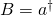and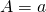is employed, such that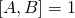.

The spectrum of solutions to the Schrödinger Equation are familiar — the first three are shown in the following plot: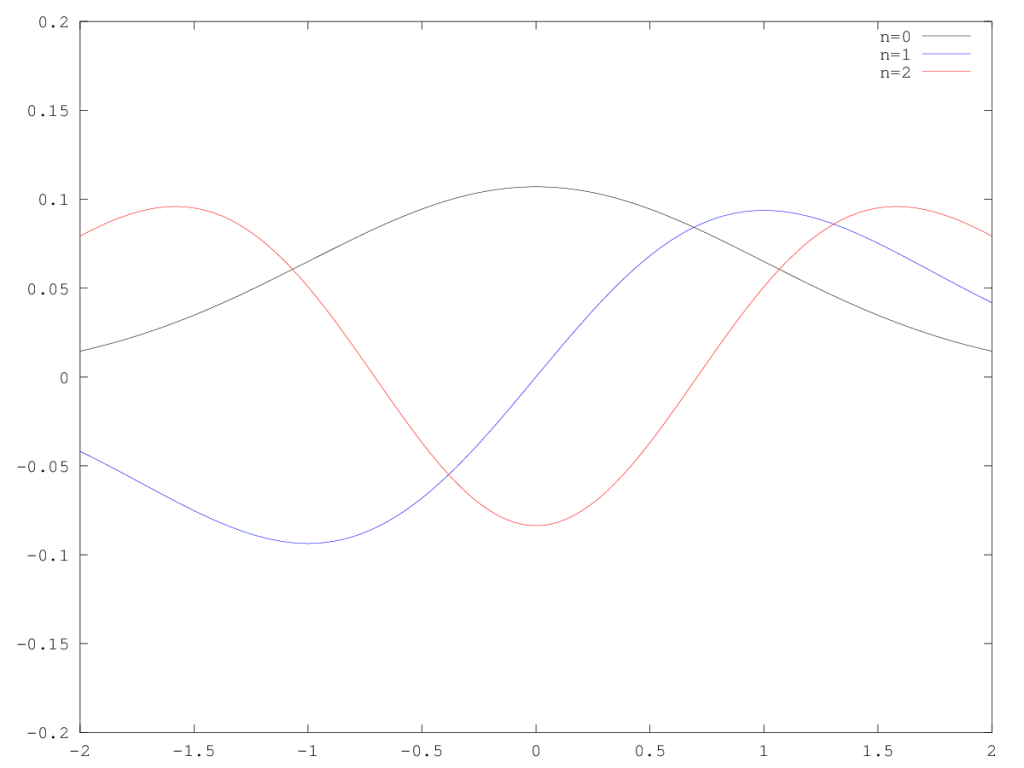### The Quartic Perturbation to the Quantum Harmonic Oscillator — Rewriting the Hamiltonian

With the above notation, we can readily incorporate a perturbation of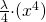into our Hamiltonian:can be normal ordered to the following result:

(1)Intuitively, we know that the result of this perturbation will be a slight change in the resulting eigenstates making up the solution.  A plot of the resulting change for an arbitrarily chosen lambda is shown below: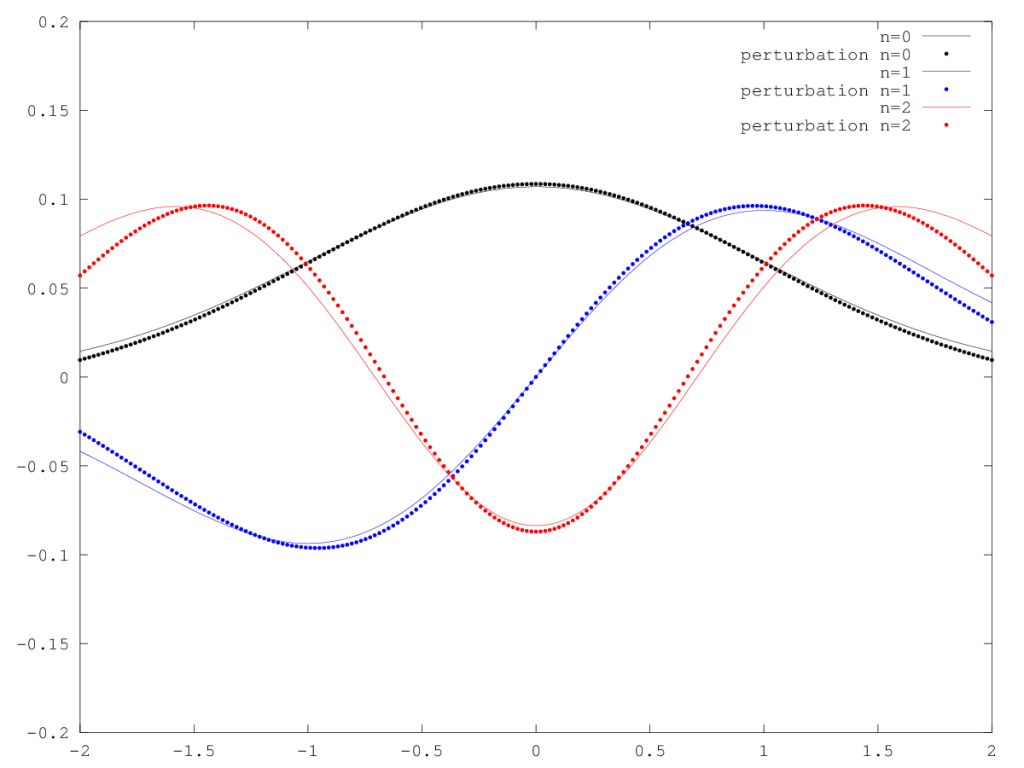### Computing the Eigenvalues to First Order ininEasy Steps

Step 1: Identify All Elements of the Lie Algebra

Elements of the Lie Algebra at first order (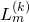where) are determined by performing commutations with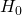and, as identified below.  At first order, terms of order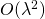are ignored, so only one commutation is required.

The first commutator:

(2)At this point, we can identify all the terms of the Lie Algebra to first order.

Nonperturbative Terms (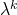where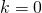)

(3)First Order Terms (where)

(4)In this representation, we see the following:

(5)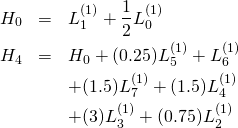This representation is complete for our purposes because it satisfies two conditions:

1.can be completely represented by terms in the algebra.
2. No two terms can be commuted to create a third non-trivial term not shown in the group.  (Remember,).

Step 2: Construct a General Lie Group Element

In principle, the Lie group element could be constructed from all terms in the Lie algebra, like so:But, by nature of the Hammard lemma, we can choose to exclude all terms that commute with.  So we construct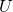as follows: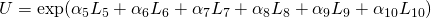This gives us 6 constants we tune in order make this Lie group element a transformation of basis between perturbed and unperturbed eigenstates.

Step 3: Use the Hammard Lemma to Compute our Lie Group Element

It is our goal to choose asuch that the following is true:

(6)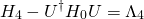where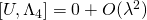where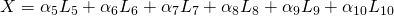.

To first order inthis simplifies to:

(7)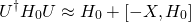Performing the commutator of Equation 7 and normal ordering, we get the following:

(8)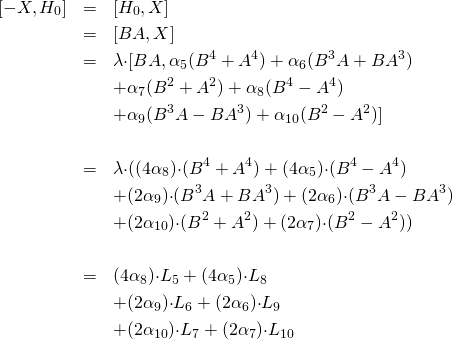Step: Tune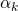so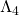is a Number Operator

From Equations 5 and 7,

(9)Now, using our knowledge thatmust commute with, we know thatcannot have terms involving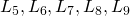or.  Thus, the alphas must be tuned such that:

(10)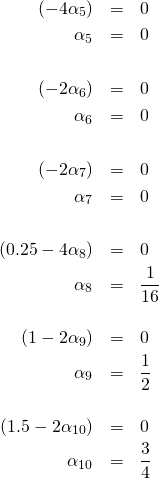Which leaves:

(11)Huzzah!  We’ve done it.  This is the perturbation to the eigenvalue introduced by the quartic term.

1. The solution came down to performing a few commutators (Step 1), expanding the Hammard Lemma (Step 3), and solving a simple system of equations, (Step).
2. It stays just that simple for all orders in.
3. It stays just that simple for all perturbations of the form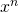Perhaps there’s more here.  Perhaps we could find a general form for the perturbation to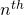order (in) to the harmonic oscillator?  Or more?